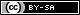# Dev Blog

 ./dev Original theme by orderedlist (CC-BY-SA) Where applicable, all content is licensed under a CC-BY-SA.# Halting Problem

The Halting Problem asks whether there exists a program that takes other programs as input and determines whether they loop forever or halt.

Assume such a program exists and call it HP.

The program HP(P,X) assumes:

• HP is a finite program
• HP stops in finite time
• HP takes as input a program, P, with input, X, both of finite length
• HP can access arbitrarily long memory such as a tape in a Turing Machine model.

Though a bit far afield from the current topic, there also needs to be constraints on the time it takes to access distant memory so as not to 'hide' computation in memory access. For example, assuming memory is a linear tape and the time to reach a distance, d, from the current location takes time proportional to d.

Consider the program SPITE:

SPITE(P) {

if (HP(P,P) reports P halts with P as input) {
while (true) {} // loop forever
}

else if (HP(P,P) reports P loops forever with P as input) {
halt // return
}

}


When we run SPITE(SPITE) (SPITE with itself as input), there are two cases:

• SPITE(SPITE) halts, in which case the first condition would have been hit, contradicting the subsequent action of looping forever.
• SPITE(SPITE) loops forever, in which case the second condition would have been hit, contradicting the subsequent action of halting.

No matter the path we take, we get a contradiction, proving the impossibility of HP existing with our given assumptions.

A quick note as it applies to statement verification.

The proof is very much the same.

To proceed via a proof by contradiction, we assume a function called Verifier, which takes in a statement and returns whether that statement is true or false.

We have to allow for functions (Verifier is one after all) and so we need to differentiate between function evaluation and writing out a statement without evaluation.

Below, the convention {.} will be used to denote the "source" of a statement rather than it's evaluation. That is, {f(s)} is the statement f(s) rather than the evaluation of the function f on statement s.

We construct the following functions:

func FunctionVerifier(f,s)
if Verifier( { f(s) } ) return True
else return False

func Spite(f)
if FunctionVerifier(f,f) return False
else return True


We then get a contradiction by asking what the return value of Spite(Spite) is.

The above is a bit sloppy because of the differentiation between function, function evaluation, statement, etc. and is why I prefer the Turing Machine model (or any more formal programming language model) but hopefully the intent should be clear.